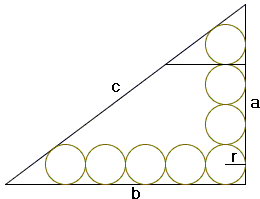## Circles Lined on the Legs of a Right Triangle: What Is This About? A Mathematical Droodle

### This applet requires Sun's Java VM 2 which your browser may perceive as a popup. Which it is not. If you want to see the applet work, visit Sun's website at https://www.java.com/en/download/index.jsp, download and install Java VM and enjoy the applet.

 What if applet does not run?

SolutionThe applet purports to suggest an extension of the following sangaku [Temple Geometry, p. 36]:

 ABC is a right-angled triangle at C, and 8 circles of the same radius r lie within the triangle and have various contacts. O1(r) touches BC and AB. O2(r) touches BC and O1(r), O3(r) touches BC and O2(r), O4(r) touches BC and O3(r), and O5(r) touches both BC and AC and also O4(r). O6(r) touches AC and O5(r), O7(r) touches AC and O6(r), and finally O8(r) touches AC and AB and O7(r). Show that the radius of the incircle of triangle ABC is equal to 3r.

(The problem appears on a surviving 1892 tablet in the Hyogo prefecture. )Draw a line parallel to the b = AC side of the triangle through the upper most point of tangency of the circles. We get two similar right triangles with legs a and (a - 6r) and inradius, say, R and r. Which gives a proportion

 (1) r/R = (a - 6r) / a = 1 - 6r/a.

For a triangle similarly obtained in the A corner we have

 r/R = (b - 8r) / b = 1 - 8r/b.

Comparing the two expressions we see that

 8a = 6b or 4a = 3b.

If, for example, a = 3 then b = 4 and the hypotenuse equals 5 giving a 3-4-5 triangle. The inradius can be found from

 R = (a + b - c)/2,

or, in this case, R = (3 + 4 - 5)/2 = 1. On the other hand, from say (1),

 r = aR / (a + 6R),

so that r = 1/3 and, indeed, R = 3r, as required.

As the applet shows (and calculations are entirely generic), any Pythagorean triple n = N2 - M2, m = 2NM, N2 + M2 leads to a right triangle along which legs may be lined (n + 1) and (m + 1) equal circles (to the total of n + m + 1) whose common radius is an integer fraction of the triangle's incircle.

### References

1. H. Fukagawa, D. Pedoe, Japanese Temple Geometry Problems, The Charles Babbage Research Center, Winnipeg, 1989

Write to:

Charles Babbage Research Center
P.O. Box 272, St. Norbert Postal Station
Winnipeg, MB